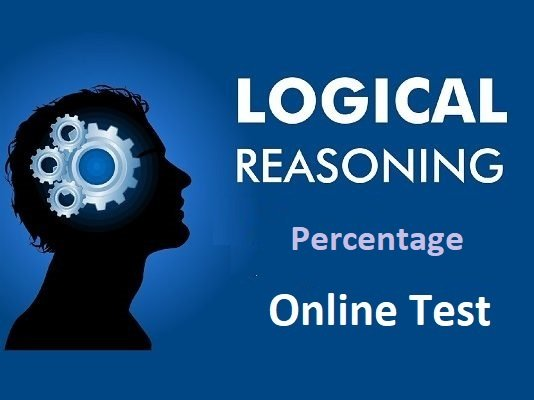# Percentage Online Test – 02

Reasoning Question Percentage questions and answers with explanations are provided for your competitive exams, placement interviews, and entrance exams.
This Reasoning Online Test contains :

• Percentage questions in both English and Hindi language.
• Selected imported 15 Percentage Questions.
• Detailed solution of each question.
0%
3

Reasoning QuestionPercentage Test - 02

1 / 15

1. If 50% of P =25\% of Q, then P = x% of Q. Find x.
यदि P का 50% = Q का 25% है, तो P = Q का x % है, तो x का मान ज्ञात करें ?

2 / 15

2. If 20% of A = 50% of B, then what percent of A is B ?
यदि A का 20 %=B का 50% है, तो A का कितना प्रतिशत B है ?

3 / 15

3. 18% of which number is equal to 12% of 75 ?

किस संख्या का 18%,75 के 12% के बराबर होगा ?

4 / 15

4. Which number is 40% less than 90% of 100?
कौन सी संख्या 100 के 90% से 40% कम होगी?

5 / 15

5. If 30% of A = 0.25 of B = 1/5 of C, then A: B : C is equal to :

यदि A का 30% = B का 0.25 = C का 1/5 है, तो A: B: C किसके बराबर है?

6 / 15

6. 0.01 is what per cent of 0.1 ?
0.1 का कितना प्रतिशत 0.01 है?

7 / 15

7. The difference of two numbers is 15% of their sum. the ratio of the larger number to the smaller num- ber is:

दो संख्याओं का अंतर, उनके योग का 15% है, तो बड़ी संख्या तथा छोटी संख्या का अनुपात ज्ञात करें?

8 / 15

8. P is six times as large as Q. The percent that Q is less than P, is: P, Q से 6 गुना बड़ा है, तो Q, P से कितना प्रतिशत छोटा है ?

9 / 15

9. 65g is what per cent of 2 kg ?
65g, 2 कि. प्रा. का कितना प्रतिशत है ?

10 / 15

10. The time duration of 2 hour 45 miuntes is what percent of day ?

2 घंटा 45 मिनट का समय, एक दिन का कितना प्रतिशत है ?

11 / 15

11. Half of 1 percent, written as a deci- mal, is:

1% का आधा दशमलव में किस प्रकार लिखा जाएगा ?

12 / 15

12. If 60% of A = 3/4 of B, then A: B is

'यदि A का 60% = B का 3/4 है, तो A : B =?

13 / 15

13. If 30% of (B - A) = 18% of (B + A) , then the ratio A: B is equal to
यदि (B-A) का 30 % = (B + A) का 18% है, तो A : B का अनुपात क्या है ?

14 / 15

14. If 90% of A= 30% of B and B is x% of A, then the value of x is
यदि A का 90%= B का 30% और B = A का x % है, तो x का मान ज्ञात करें ?

15 / 15

15. One-third of 1206 is what per cent of 134 ?

1206 का एक-तिहाई, 134 का कितने प्रतिशत है ?